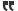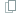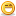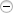# Thread: The easy way to calc probabilities for your deck designs

1. Very cool and handy tool, thanks for sharing!

One thing to point out though would be that the Cumulative Probability: P(X > x) displays the correct value and not the Hypergeometric Probability: P(X = x). Don't know why though, since the Hypergeometric Probability should be the one that matters as far as I understand.Reply With Quote

2.Originally Posted by GondorianDotComI've finished v0.3 of the deck simulator: Give it a try

Note: You must enter your deck string in the same format that you see when you make your deck with Orca's deckbuilder.

Sorry for hijacking this thread. I will make another if necessary.
The simulator looks promising. One thing is that it work in a tandem with the Orca's deckbuilder, i.e. build the deck with the builder then feed its output to the simulator. The Orca's database hasn't been updated since 1.25. Are there news with regards to updating the Orca's database to 1.277 and subsequent releases?Reply With Quote

3. I updated his deck builder for him, but I think he is doing his own update now too.

In the mean time, go here:

Enjoy!

p.s. I do not think my simulator will work for new cards and it is kinda redundant when people can use actual probabilities with calculators. It was just a bit of fun really, and one of my initial ways of trying to add value here. Now I have the articles, deckbuilder, leagues, tournaments, so I don't really promote the simulator any more. Glad you like it though.Reply With Quote

4.Originally Posted by GondorianDotComI updated his deck builder for him, but I think he is doing his own update now too.

In the mean time, go here:

Enjoy!

p.s. I do not think my simulator will work for new cards and it is kinda redundant when people can use actual probabilities with calculators. It was just a bit of fun really, and one of my initial ways of trying to add value here. Now I have the articles, deckbuilder, leagues, tournaments, so I don't really promote the simulator any more. Glad you like it though.
ic, the simulator is a useful tool. Lay out the probability of all the cards of the deck in one neat page. Instead of manually calculating each card individually.

Appreciate the 1.27 deck builder. Thanks for updating and maintaining it.Reply With Quote

5.Originally Posted by shannong3 - How to calculate OR probabilities

Per the 3rd bullet above, it's very easy to calculate the probability of card A OR card B, either on the same turn or different turns. In this case, you simply ADD the two probabilities together, then divide by 2.

(Px + Py) / 2 = PxORy
I don't think this is correct. The standard formula is: P(x OR y) = P(x) + P(y) - P(x AND y), which in case of independent events x and y reduces to P(x) + P(y) - P(x)P(y).

Just taking the average of the probabilities can never work, not even as approximation, as P(x OR y) should always be larger than both P(x) and P(y)Reply With Quote

6. I'm a human being I'm just gonna use meh gut!! My... "intuition".Reply With Quote

7.Originally Posted by SprinklesI'm a human being I'm just gonna use meh gut!! My... "intuition".
necro troll!Reply With Quote

8. 3 - How to calculate OR probabilities

Per the 3rd bullet above, it's very easy to calculate the probability of card A OR card B, either on the same turn or different turns. In this case, you simply ADD the two probabilities together, then divide by 2.

(Px + Py) / 2 = PxORy
I am not sure if this works. Let's take an example to see if works. I have Kristofferx 4 and Birgittex 4 in a deck. I want to have at least one of these in my hand at the beginning of the game when I go first.

So essentially, I am saying there are 8 cards in my deck (with cost of 1) and I need at least one of which drawn at the beginning of the game. So I put 39/8/6/1, which gives 77%.

Alternatively you can phrase such that, I need either one Kristofferat beginning OR Birgitteat beginning.

So I put 39/4/6/1, which gives 50% for each. If you add two and divide by ２, it simply goes back to 50%.

It's been so long since I did statistics and it was my least favorite class, but I believe issue here is you have to subtract something like overlap of the two probability. So Px + Py - PxANDPy, which gives 75% and not quite 77% so I must be doing something wrong here, but definitely closer...

In any event, I feel like simply adding and dividing by 2 may not work.

Am I missing or just too confused here? Could someone clarify this for me?Reply With Quote

9. When you look at P>=1, you are looking at the provability of getting AT LEAST 1, not exactly 1 positive result.

So for 39/8/6/1, you have 77% chances of getting at least 1 Kris OR 1 Birgette. (you'll get only one of them 42% of the time and 2+ in 36% of your games)

If you do 39/4/6/1 twice, you're calculating the chances of getting at least 1 Kris AND at least 1 Birgette. Individually, you will draw one in 40% of your opening hands and a mix of 2+ 1cc allies 10% of the time (50% odds of getting at least 1). So 75% of getting at least one of each.

Add the odds of getting at least a Bad Santa(39/4/7/1), 56%, to get the probability of pulling a Santa Bomb (56%*77% = 43%) when going first (so 43%*50% = 22% of total games, not accounting the probability that your opponent might play a 1cc ally).Reply With Quote

10. Hi,

I think we should apply hypergeometric cummulative distribution instead of single hypergeometric distribution. If single hypergeometric distribution is correct considering that we have 4 of the same card in our deck then 3 turns before the end the probability should be 100% of having 2 of them. But using the single distribution we obtain:

Hypergeometric Probability: P(X = 2)
Turn 1 12,66%
Turn 2 15,83%
Turn 3 19,04%
Turn 4 22,21%
Turn 5 25,28%
Turn 6 28,17%
Turn 7 30,82%
Turn 8 33,19%
Turn 9 35,23%
Turn 10 36,91%
Turn 11 38,20%
Turn 12 39,06%
Turn 13 39,50%
Turn 14 39,50%
Turn 15 39,06%
Turn 16 38,20%
Turn 17 36,91%
Turn 18 35,23%
Turn 19 33,19%
Turn 20 30,82%
Turn 21 28,17%
Turn 22 25,28%
Turn 23 22,21%
Turn 24 19,04%
Turn 25 15,83%
Turn 26 12,66%
Turn 27 9,63%
Turn 28 6,82%
Turn 29 4,34%
Turn 30 2,30%
Turn 31 0,81%
Turn 32 0,00%

In this turn we have 6 + 32 cards we should have 100% percentage to have 2 identical cards, the problem of this distribution is that we are obtaining less percentage from turn 15 because we can have more than 2 cards and that is why is translated as less probability with this distribution.

However with hypergeometric cumulative distribution we can know the probability of having 2 or more cards-

Cumulative Probability: P(X > or = 2)

Turn 1 14,07%
Turn 2 18,03%
Turn 3 22,26%
Turn 4 26,70%
Turn 5 31,29%
Turn 6 35,99%
Turn 7 40,73%
Turn 8 45,47%
Turn 9 50,17%
Turn 10 54,78%
Turn 11 59,28%
Turn 12 63,62%
Turn 13 67,78%
Turn 14 71,73%
Turn 15 75,45%
Turn 16 78,92%
Turn 17 82,13%
Turn 18 85,06%
Turn 19 87,72%
Turn 20 90,09%
Turn 21 92,18%
Turn 22 93,98%
Turn 23 95,51%
Turn 24 96,78%
Turn 25 97,80%
Turn 26 98,60%
Turn 27 99,18%
Turn 28 99,58%
Turn 29 99,83%
Turn 30 99,96%
Turn 31 100,00% In this turn we have draw a total of 6 + 31 cards, in this turn for sure that we have twice the card.

In excel we can use the following formula

1-(HYPGEOM.DIST(X1;X2;X3;X4-1;TRUE)-HYPGEOM.DIST(\$D\$12;F15;\$D\$13;\$D\$11-1;FALSE))

X1: How many times do you need that card to appear?
X2: number of turn the first turn will be 7 (6+1 card), the second 8......
X3: How many cards of that you have on your deck?
X4: total number of cards including hero, the -1 is to consider that.

Sorry for my englishReply With Quote

####Posting Permissions

• You may not post new threads
• You may not post replies
• You may not post attachments
• You may not edit your posts
•